# Selina Solutions Concise Maths Class 7 Chapter 6: Ratio and Proportion (Including Sharing in a Ratio) Exercise 6A

Selina Solutions Concise Maths Class 7 Chapter 6 Ratio and Proportion (Including Sharing in a Ratio) Exercise 6A help students understand the fundamental concepts covered in this chapter. Conversion of a fractional number into a whole number ratio and division of a given quantity into a given ratio are the topics, which are explained in this exercise. For better conceptual knowledge, students can make use of Selina Solutions Concise Maths Class 7 Chapter 6 Ratio and Proportion (Including Sharing in a Ratio) Exercise 6A PDF from the links, which are provided below.

## Selina Solutions Concise Maths Class 7 Chapter 6: Ratio and Proportion (Including Sharing in a Ratio) Exercise 6A Download PDF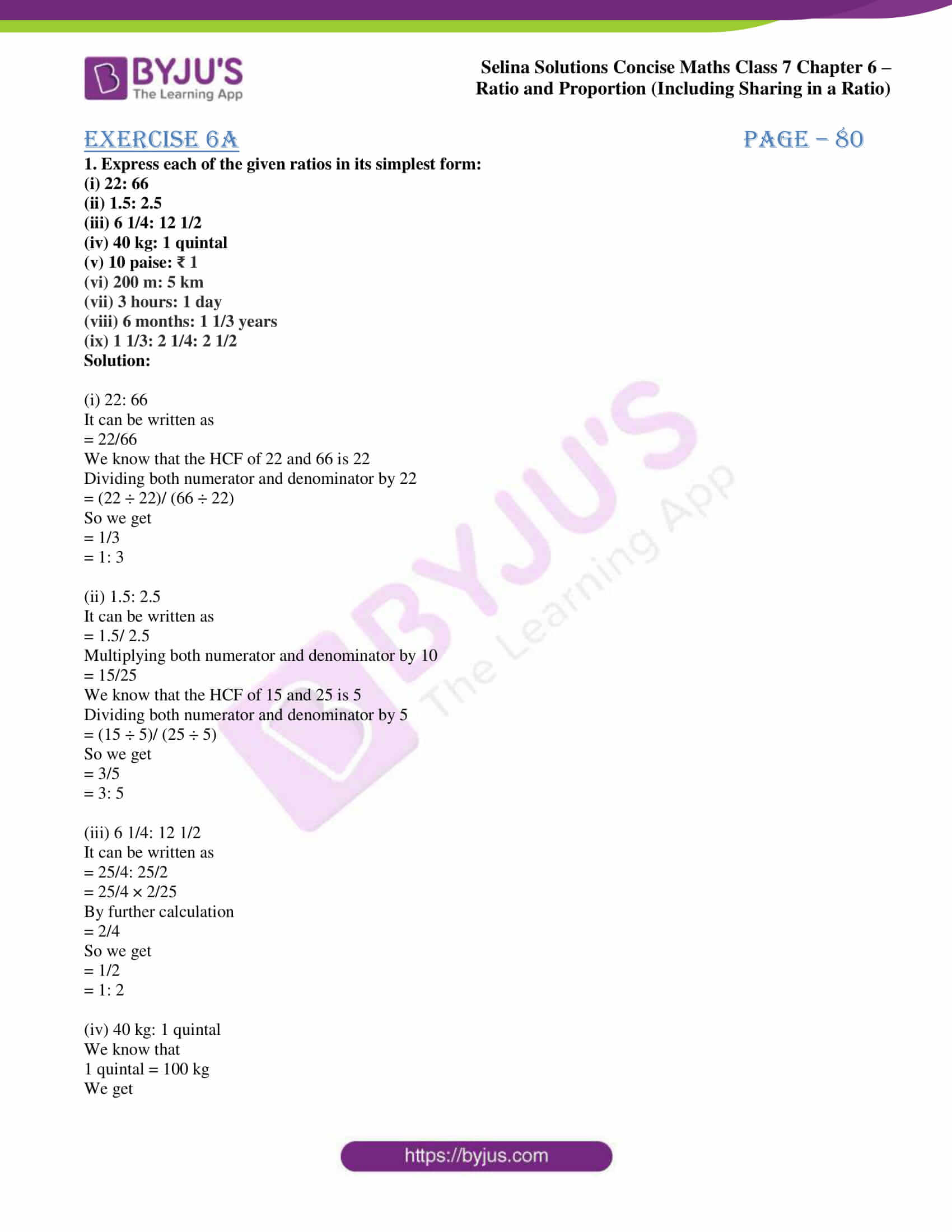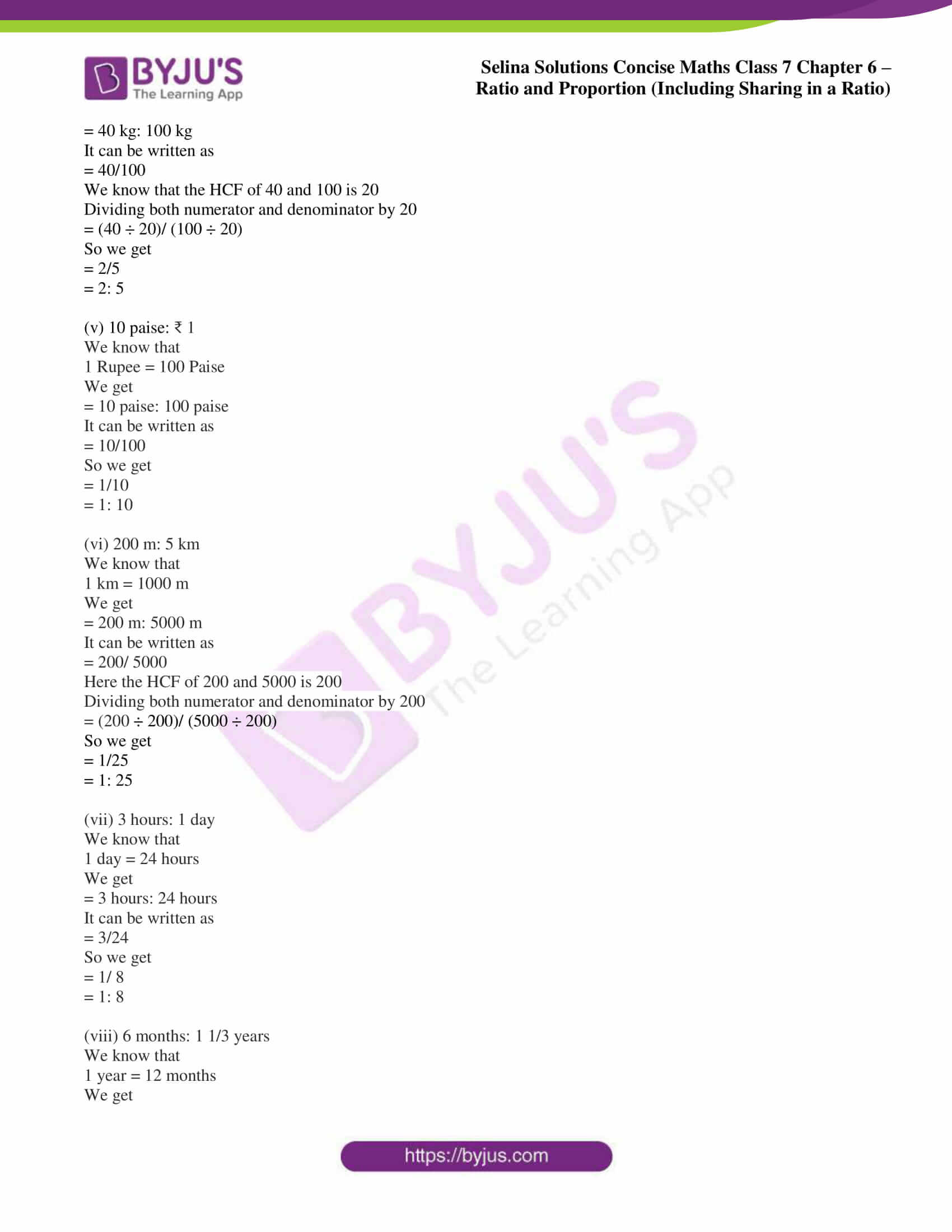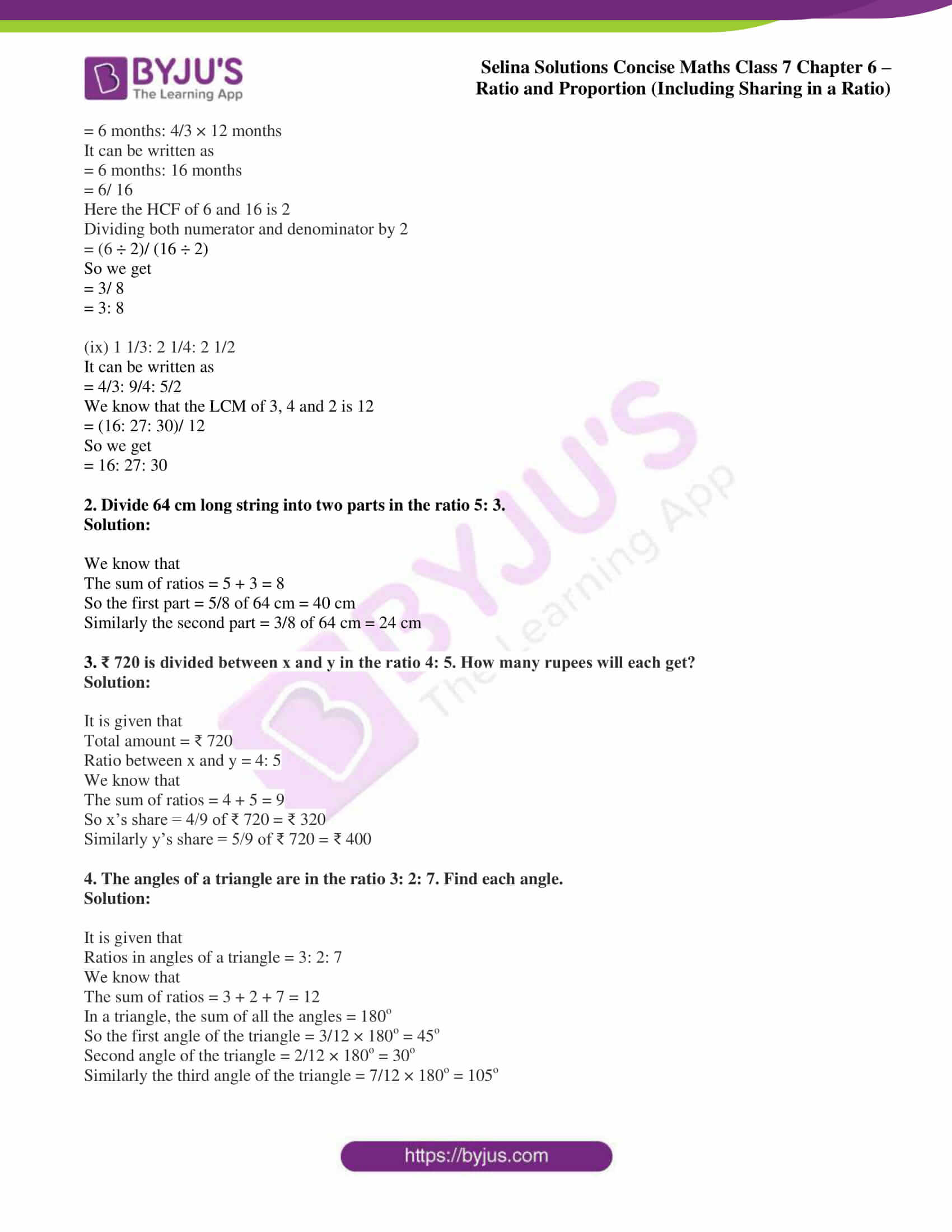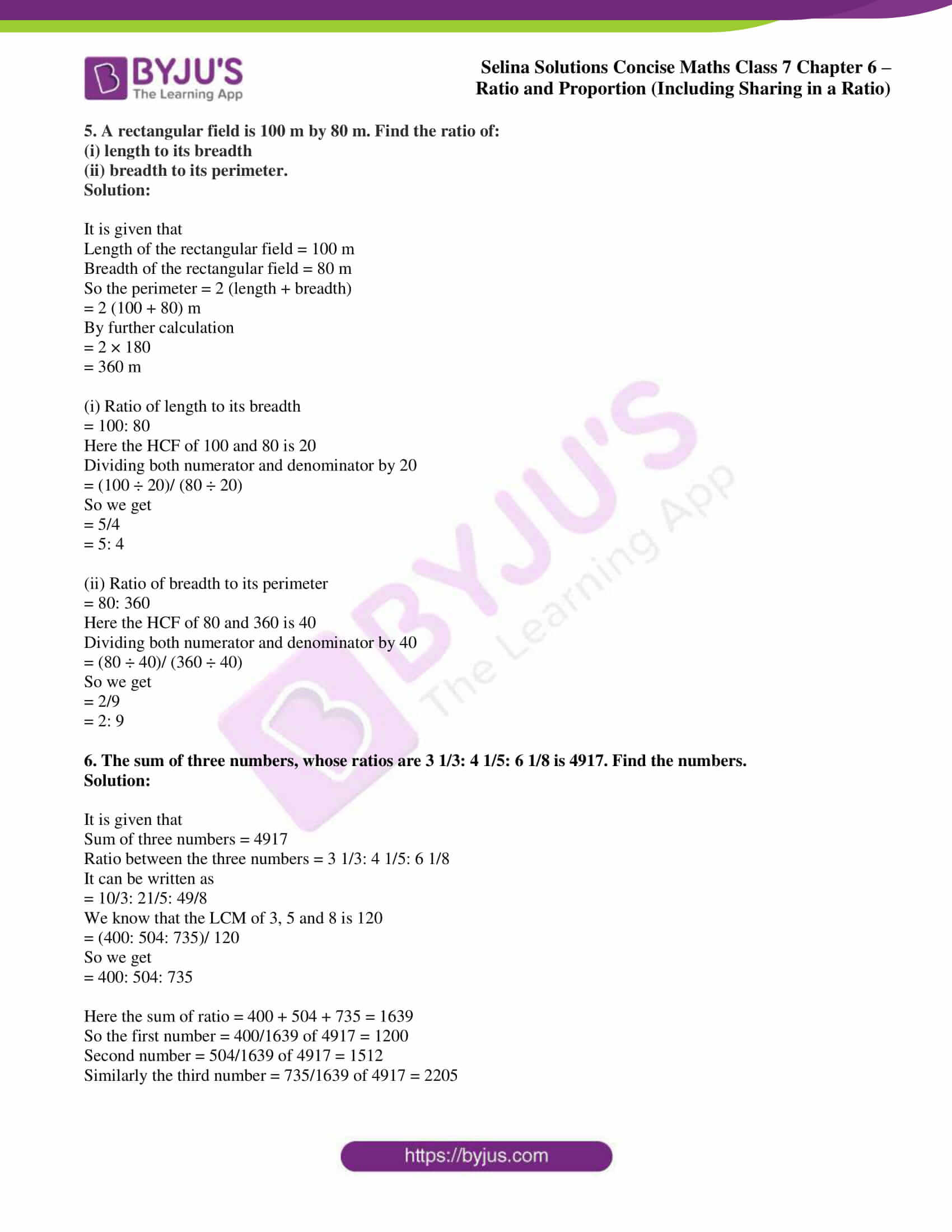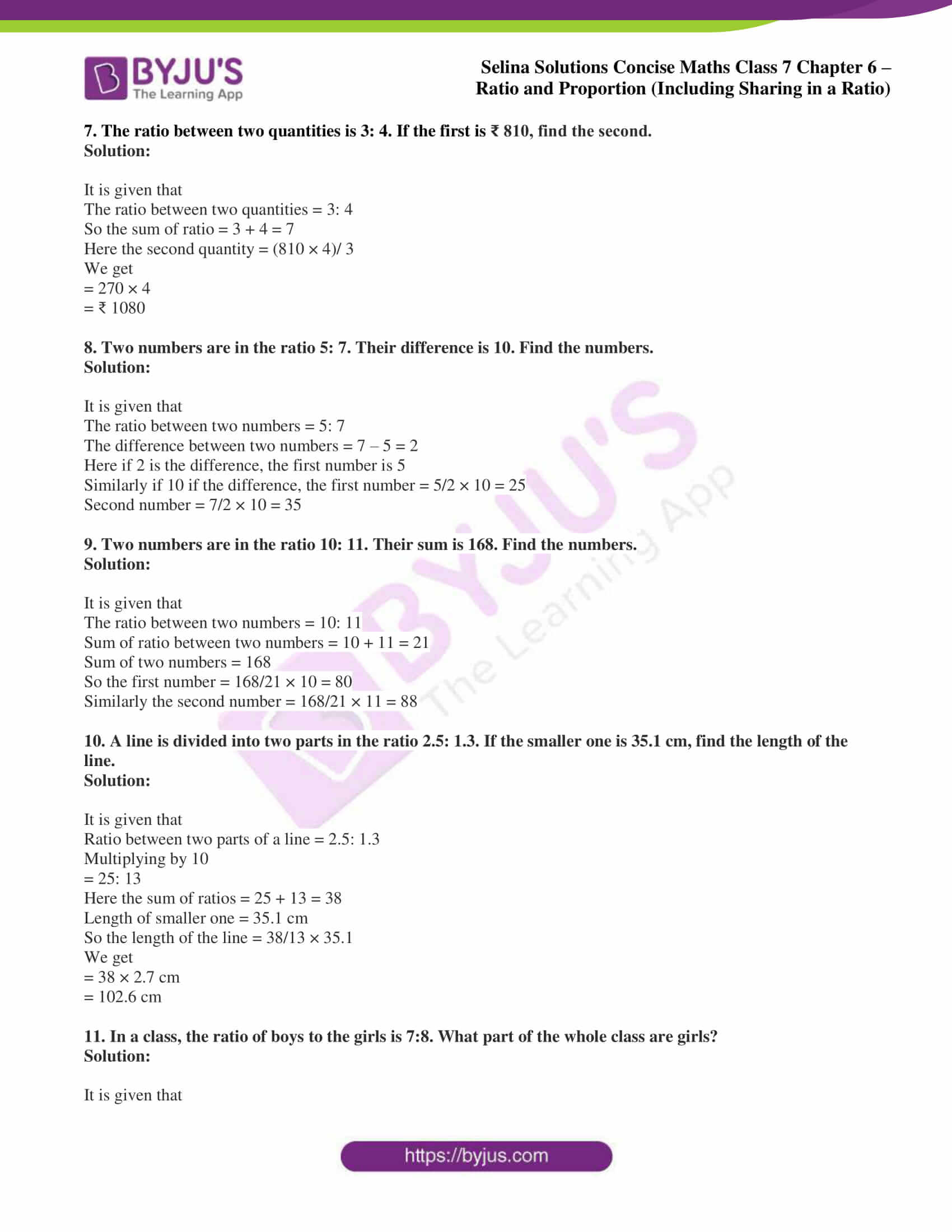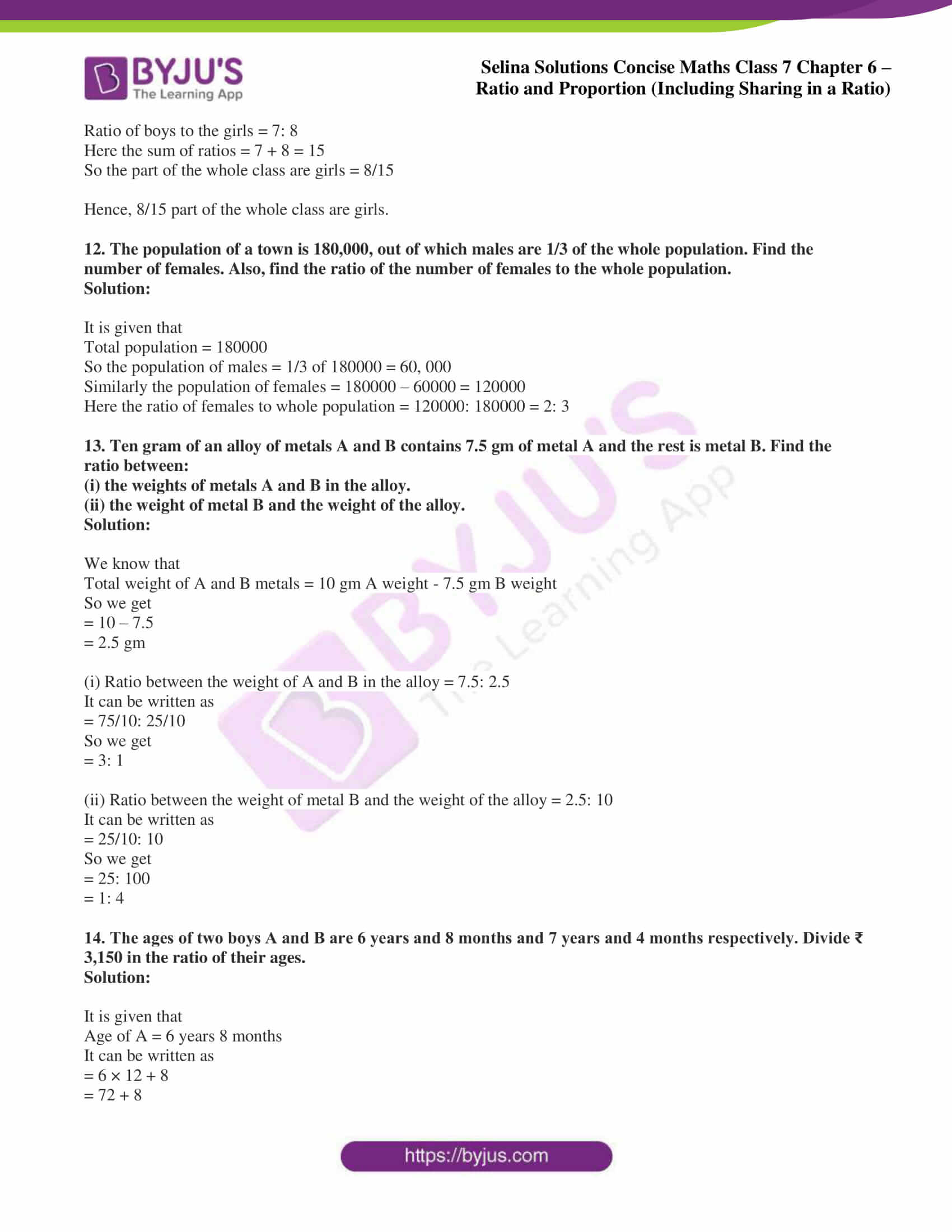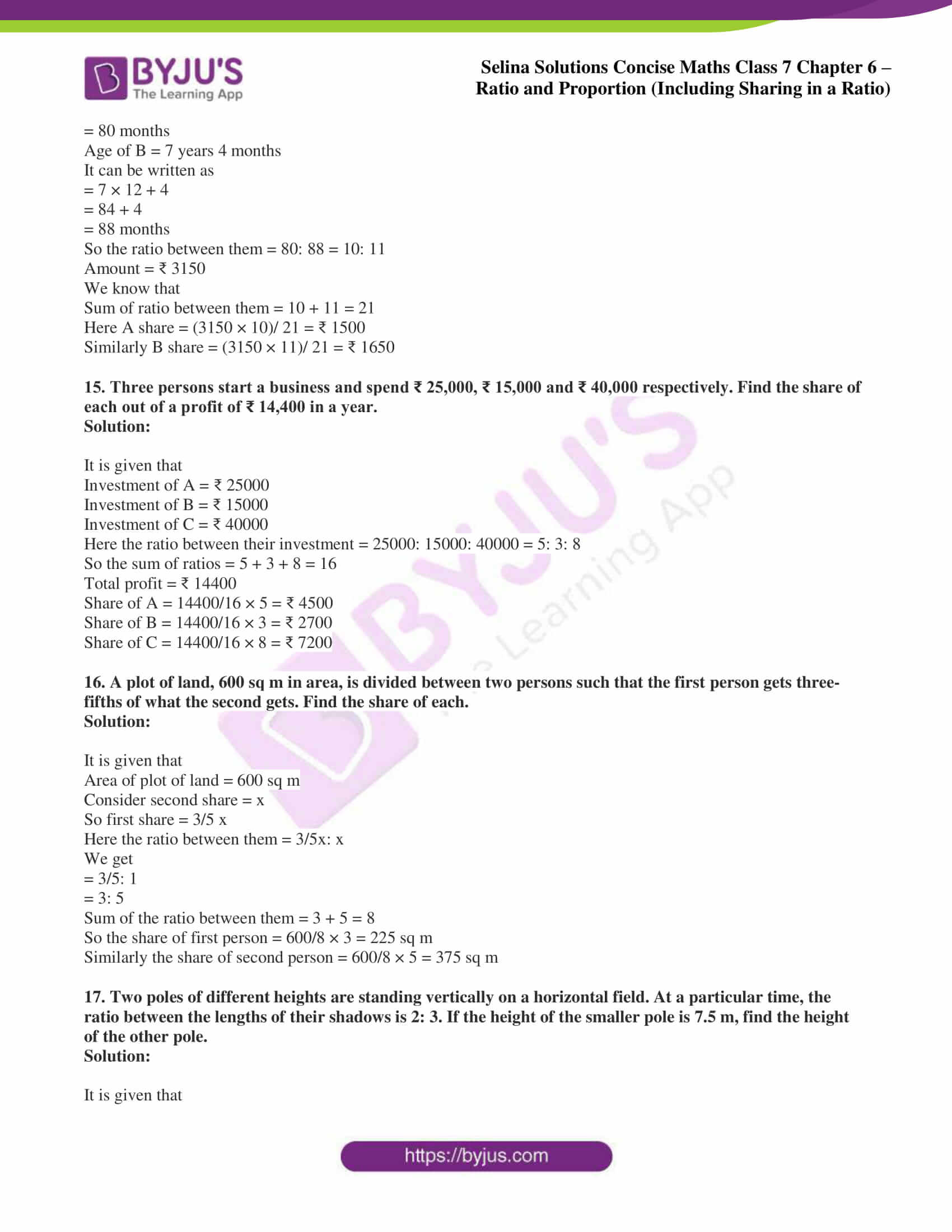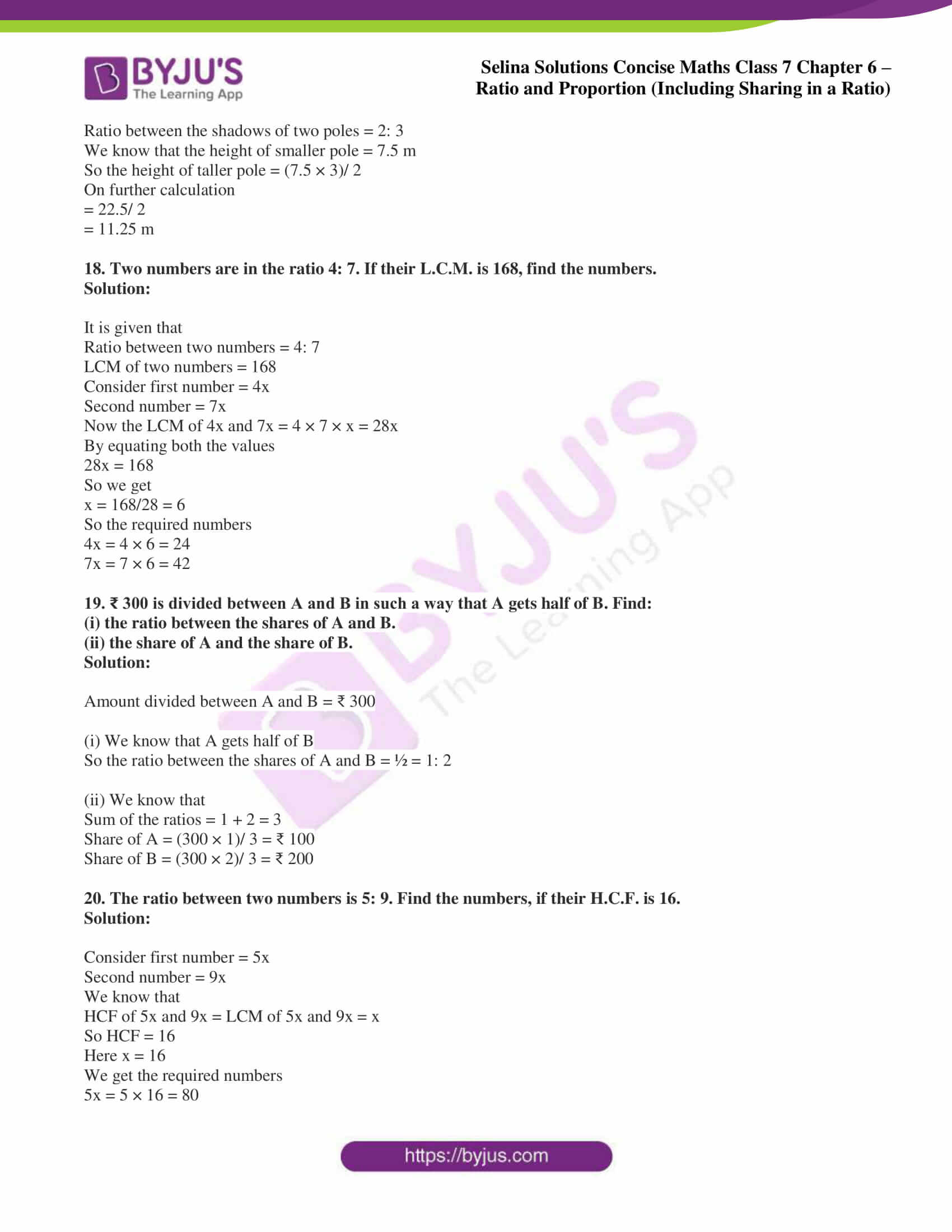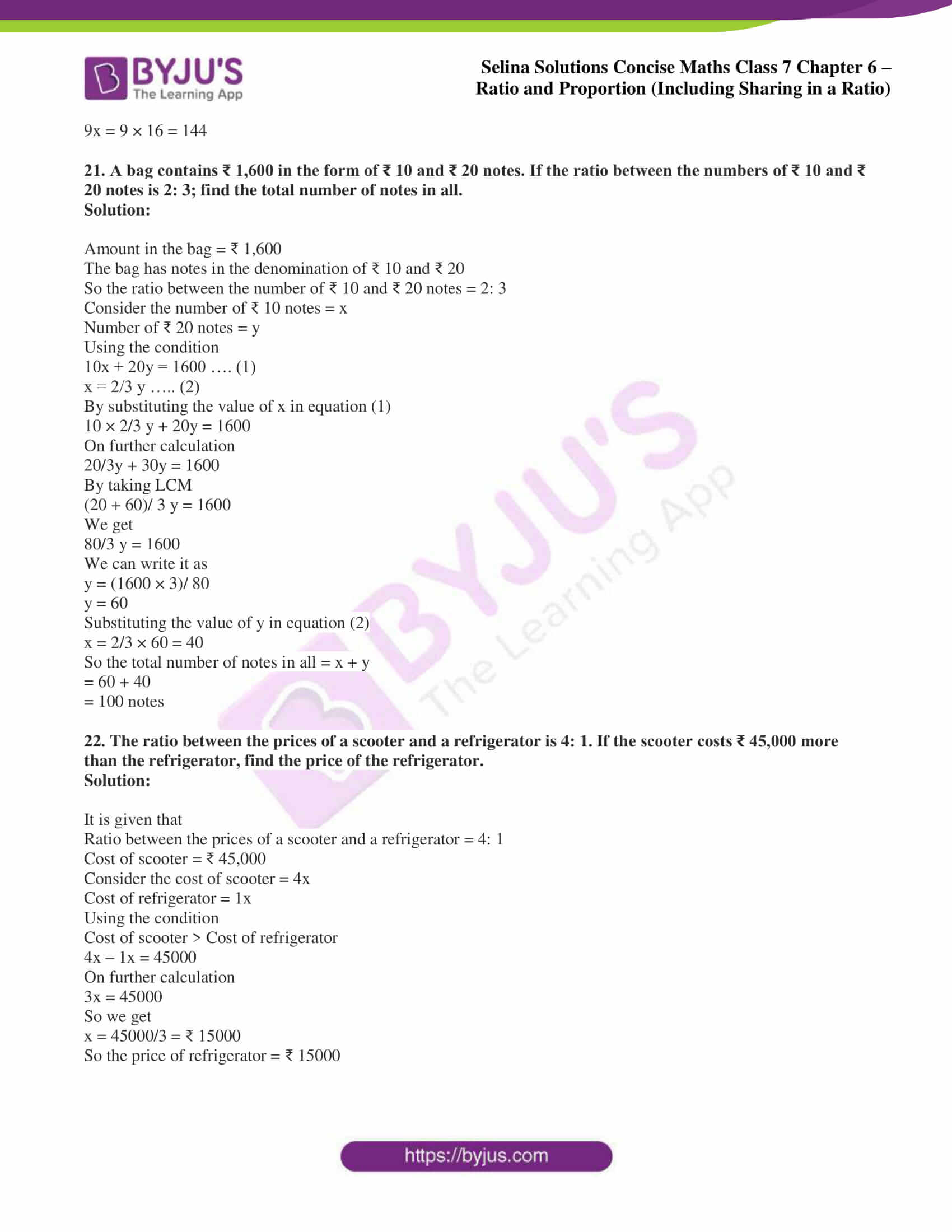### Access Selina Solutions Concise Maths Class 7 Chapter 6: Ratio and Proportion (Including Sharing in a Ratio) Exercise 6A

1. Express each of the given ratios in its simplest form:

(i) 22: 66

(ii) 1.5: 2.5

(iii) 6 1/4: 12 1/2

(iv) 40 kg: 1 quintal

(v) 10 paise: ₹ 1

(vi) 200 m: 5 km

(vii) 3 hours: 1 day

(viii) 6 months: 1 1/3 years

(ix) 1 1/3: 2 1/4: 2 1/2

Solution:

(i) 22: 66

It can be written as

= 22/66

We know that the HCF of 22 and 66 is 22

Dividing both numerator and denominator by 22

= (22 ÷ 22)/ (66 ÷ 22)

So we get

= 1/3

= 1: 3

(ii) 1.5: 2.5

It can be written as

= 1.5/ 2.5

Multiplying both numerator and denominator by 10

= 15/25

We know that the HCF of 15 and 25 is 5

Dividing both numerator and denominator by 5

= (15 ÷ 5)/ (25 ÷ 5)

So we get

= 3/5

= 3: 5

(iii) 6 1/4: 12 1/2

It can be written as

= 25/4: 25/2

= 25/4 × 2/25

By further calculation

= 2/4

So we get

= 1/2

= 1: 2

(iv) 40 kg: 1 quintal

We know that

1 quintal = 100 kg

We get

= 40 kg: 100 kg

It can be written as

= 40/100

We know that the HCF of 40 and 100 is 20

Dividing both numerator and denominator by 20

= (40 ÷ 20)/ (100 ÷ 20)

So we get

= 2/5

= 2: 5

(v) 10 paise: ₹ 1

We know that

1 Rupee = 100 Paise

We get

= 10 paise: 100 paise

It can be written as

= 10/100

So we get

= 1/10

= 1: 10

(vi) 200 m: 5 km

We know that

1 km = 1000 m

We get

= 200 m: 5000 m

It can be written as

= 200/ 5000

Here the HCF of 200 and 5000 is 200

Dividing both numerator and denominator by 200

= (200 ÷ 200)/ (5000 ÷ 200)

So we get

= 1/25

= 1: 25

(vii) 3 hours: 1 day

We know that

1 day = 24 hours

We get

= 3 hours: 24 hours

It can be written as

= 3/24

So we get

= 1/ 8

= 1: 8

(viii) 6 months: 1 1/3 years

We know that

1 year = 12 months

We get

= 6 months: 4/3 × 12 months

It can be written as

= 6 months: 16 months

= 6/ 16

Here the HCF of 6 and 16 is 2

Dividing both numerator and denominator by 2

= (6 ÷ 2)/ (16 ÷ 2)

So we get

= 3/ 8

= 3: 8

(ix) 1 1/3: 2 1/4: 2 1/2

It can be written as

= 4/3: 9/4: 5/2

We know that the LCM of 3, 4 and 2 is 12

= (16: 27: 30)/ 12

So we get

= 16: 27: 30

2. Divide 64 cm long string into two parts in the ratio 5: 3.

Solution:

We know that

The sum of ratios = 5 + 3 = 8

So the first part = 5/8 of 64 cm = 40 cm

Similarly the second part = 3/8 of 64 cm = 24 cm

3. ₹ 720 is divided between x and y in the ratio 4: 5. How many rupees will each get?

Solution:

It is given that

Total amount = ₹ 720

Ratio between x and y = 4: 5

We know that

The sum of ratios = 4 + 5 = 9

So x’s share = 4/9 of ₹ 720 = ₹ 320

Similarly y’s share = 5/9 of ₹ 720 = ₹ 400

4. The angles of a triangle are in the ratio 3: 2: 7. Find each angle.

Solution:

It is given that

Ratios in angles of a triangle = 3: 2: 7

We know that

The sum of ratios = 3 + 2 + 7 = 12

In a triangle, the sum of all the angles = 180o

So the first angle of the triangle = 3/12 × 180o = 45o

Second angle of the triangle = 2/12 × 180o = 30o

Similarly the third angle of the triangle = 7/12 × 180o = 105o

5. A rectangular field is 100 m by 80 m. Find the ratio of:

Solution:

It is given that

Length of the rectangular field = 100 m

Breadth of the rectangular field = 80 m

So the perimeter = 2 (length + breadth)

= 2 (100 + 80) m

By further calculation

= 2 × 180

= 360 m

(i) Ratio of length to its breadth

= 100: 80

Here the HCF of 100 and 80 is 20

Dividing both numerator and denominator by 20

= (100 ÷ 20)/ (80 ÷ 20)

So we get

= 5/4

= 5: 4

(ii) Ratio of breadth to its perimeter

= 80: 360

Here the HCF of 80 and 360 is 40

Dividing both numerator and denominator by 40

= (80 ÷ 40)/ (360 ÷ 40)

So we get

= 2/9

= 2: 9

6. The sum of three numbers, whose ratios are 3 1/3: 4 1/5: 6 1/8 is 4917. Find the numbers.

Solution:

It is given that

Sum of three numbers = 4917

Ratio between the three numbers = 3 1/3: 4 1/5: 6 1/8

It can be written as

= 10/3: 21/5: 49/8

We know that the LCM of 3, 5 and 8 is 120

= (400: 504: 735)/ 120

So we get

= 400: 504: 735

Here the sum of ratio = 400 + 504 + 735 = 1639

So the first number = 400/1639 of 4917 = 1200

Second number = 504/1639 of 4917 = 1512

Similarly the third number = 735/1639 of 4917 = 2205

7. The ratio between two quantities is 3: 4. If the first is ₹ 810, find the second.

Solution:

It is given that

The ratio between two quantities = 3: 4

So the sum of ratio = 3 + 4 = 7

Here the second quantity = (810 × 4)/ 3

We get

= 270 × 4

= ₹ 1080

8. Two numbers are in the ratio 5: 7. Their difference is 10. Find the numbers.

Solution:

It is given that

The ratio between two numbers = 5: 7

The difference between two numbers = 7 – 5 = 2

Here if 2 is the difference, the first number is 5

Similarly if 10 if the difference, the first number = 5/2 × 10 = 25

Second number = 7/2 × 10 = 35

9. Two numbers are in the ratio 10: 11. Their sum is 168. Find the numbers.

Solution:

It is given that

The ratio between two numbers = 10: 11

Sum of ratio between two numbers = 10 + 11 = 21

Sum of two numbers = 168

So the first number = 168/21 × 10 = 80

Similarly the second number = 168/21 × 11 = 88

10. A line is divided into two parts in the ratio 2.5: 1.3. If the smaller one is 35.1 cm, find the length of the line.

Solution:

It is given that

Ratio between two parts of a line = 2.5: 1.3

Multiplying by 10

= 25: 13

Here the sum of ratios = 25 + 13 = 38

Length of smaller one = 35.1 cm

So the length of the line = 38/13 × 35.1

We get

= 38 × 2.7 cm

= 102.6 cm

11. In a class, the ratio of boys to the girls is 7:8. What part of the whole class are girls?

Solution:

It is given that

Ratio of boys to the girls = 7: 8

Here the sum of ratios = 7 + 8 = 15

So the part of the whole class are girls = 8/15

Hence, 8/15 part of the whole class are girls.

12. The population of a town is 180,000, out of which males are 1/3 of the whole population. Find the number of females. Also, find the ratio of the number of females to the whole population.

Solution:

It is given that

Total population = 180000

So the population of males = 1/3 of 180000 = 60, 000

Similarly the population of females = 180000 – 60000 = 120000

Here the ratio of females to whole population = 120000: 180000 = 2: 3

13. Ten gram of an alloy of metals A and B contains 7.5 gm of metal A and the rest is metal B. Find the ratio between:

(i) the weights of metals A and B in the alloy.

(ii) the weight of metal B and the weight of the alloy.

Solution:

We know that

Total weight of A and B metals = 10 gm A weight – 7.5 gm B weight

So we get

= 10 – 7.5

= 2.5 gm

(i) Ratio between the weight of A and B in the alloy = 7.5: 2.5

It can be written as

= 75/10: 25/10

So we get

= 3: 1

(ii) Ratio between the weight of metal B and the weight of the alloy = 2.5: 10

It can be written as

= 25/10: 10

So we get

= 25: 100

= 1: 4

14. The ages of two boys A and B are 6 years and 8 months and 7 years and 4 months respectively. Divide ₹ 3,150 in the ratio of their ages.

Solution:

It is given that

Age of A = 6 years 8 months

It can be written as

= 6 × 12 + 8

= 72 + 8

= 80 months

Age of B = 7 years 4 months

It can be written as

= 7 × 12 + 4

= 84 + 4

= 88 months

So the ratio between them = 80: 88 = 10: 11

Amount = ₹ 3150

We know that

Sum of ratio between them = 10 + 11 = 21

Here A share = (3150 × 10)/ 21 = ₹ 1500

Similarly B share = (3150 × 11)/ 21 = ₹ 1650

15. Three persons start a business and spend ₹ 25,000, ₹ 15,000 and ₹ 40,000 respectively. Find the share of each out of a profit of ₹ 14,400 in a year.

Solution:

It is given that

Investment of A = ₹ 25000

Investment of B = ₹ 15000

Investment of C = ₹ 40000

Here the ratio between their investment = 25000: 15000: 40000 = 5: 3: 8

So the sum of ratios = 5 + 3 + 8 = 16

Total profit = ₹ 14400

Share of A = 14400/16 × 5 = ₹ 4500

Share of B = 14400/16 × 3 = ₹ 2700

Share of C = 14400/16 × 8 = ₹ 7200

16. A plot of land, 600 sq m in area, is divided between two persons such that the first person gets three-fifths of what the second gets. Find the share of each.

Solution:

It is given that

Area of plot of land = 600 sq m

Consider second share = x

So first share = 3/5 x

Here the ratio between them = 3/5x: x

We get

= 3/5: 1

= 3: 5

Sum of the ratio between them = 3 + 5 = 8

So the share of first person = 600/8 × 3 = 225 sq m

Similarly the share of second person = 600/8 × 5 = 375 sq m

17. Two poles of different heights are standing vertically on a horizontal field. At a particular time, the ratio between the lengths of their shadows is 2: 3. If the height of the smaller pole is 7.5 m, find the height of the other pole.

Solution:

It is given that

Ratio between the shadows of two poles = 2: 3

We know that the height of smaller pole = 7.5 m

So the height of taller pole = (7.5 × 3)/ 2

On further calculation

= 22.5/ 2

= 11.25 m

18. Two numbers are in the ratio 4: 7. If their L.C.M. is 168, find the numbers.

Solution:

It is given that

Ratio between two numbers = 4: 7

LCM of two numbers = 168

Consider first number = 4x

Second number = 7x

Now the LCM of 4x and 7x = 4 × 7 × x = 28x

By equating both the values

28x = 168

So we get

x = 168/28 = 6

So the required numbers

4x = 4 × 6 = 24

7x = 7 × 6 = 42

19. ₹ 300 is divided between A and B in such a way that A gets half of B. Find:

(i) the ratio between the shares of A and B.

(ii) the share of A and the share of B.

Solution:

Amount divided between A and B = ₹ 300

(i) We know that A gets half of B

So the ratio between the shares of A and B = ½ = 1: 2

(ii) We know that

Sum of the ratios = 1 + 2 = 3

Share of A = (300 × 1)/ 3 = ₹ 100

Share of B = (300 × 2)/ 3 = ₹ 200

20. The ratio between two numbers is 5: 9. Find the numbers, if their H.C.F. is 16.

Solution:

Consider first number = 5x

Second number = 9x

We know that

HCF of 5x and 9x = LCM of 5x and 9x = x

So HCF = 16

Here x = 16

We get the required numbers

5x = 5 × 16 = 80

9x = 9 × 16 = 144

21. A bag contains ₹ 1,600 in the form of ₹ 10 and ₹ 20 notes. If the ratio between the numbers of ₹ 10 and ₹ 20 notes is 2: 3; find the total number of notes in all.

Solution:

Amount in the bag = ₹ 1,600

The bag has notes in the denomination of ₹ 10 and ₹ 20

So the ratio between the number of ₹ 10 and ₹ 20 notes = 2: 3

Consider the number of ₹ 10 notes = x

Number of ₹ 20 notes = y

Using the condition

10x + 20y = 1600 …. (1)

x = 2/3 y ….. (2)

By substituting the value of x in equation (1)

10 × 2/3 y + 20y = 1600

On further calculation

20/3y + 30y = 1600

By taking LCM

(20 + 60)/ 3 y = 1600

We get

80/3 y = 1600

We can write it as

y = (1600 × 3)/ 80

y = 60

Substituting the value of y in equation (2)

x = 2/3 × 60 = 40

So the total number of notes in all = x + y

= 60 + 40

= 100 notes

22. The ratio between the prices of a scooter and a refrigerator is 4: 1. If the scooter costs ₹ 45,000 more than the refrigerator, find the price of the refrigerator.

Solution:

It is given that

Ratio between the prices of a scooter and a refrigerator = 4: 1

Cost of scooter = ₹ 45,000

Consider the cost of scooter = 4x

Cost of refrigerator = 1x

Using the condition

Cost of scooter > Cost of refrigerator

4x – 1x = 45000

On further calculation

3x = 45000

So we get

x = 45000/3 = ₹ 15000

So the price of refrigerator = ₹ 15000